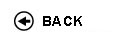Politecnico di Torino
Academic Year 2015/16
05QPWLZ, 05QPWJM, 05QPWLI, 05QPWLN, 05QPWLS, 05QPWLX, 05QPWMA, 05QPWMB, 05QPWMK, 05QPWMN, 05QPWPI, 05QPWPL
Physics II - Talenti
1st degree and Bachelor-level of the Bologna process in Aerospace Engineering - Torino
1st degree and Bachelor-level of the Bologna process in Mechanical Engineering - Torino
1st degree and Bachelor-level of the Bologna process in Automotive Engineering - Torino
Espandi...
 Teacher Status SSD Les Ex Lab Tut Years teaching Allia Paolo Maria Eugenio Icilio100 0 0 0 2
 SSD CFU Activities Area context FIS/01FIS/03 55 A - Di baseA - Di base Fisica e chimicaFisica e chimica
 Subject fundamentals Aim of the course (1st semester, 2nd year) is to provide the students of Electronic Engineering and Physical Engineering with the theoretical concepts to be used in all courses of the following semesters. This is therefore a pivotal course for the ensuing career of an electronic engineer and a physics engineer. The course is divided in two sections: in the first one (Classical Electromagnetism), fundamental subjects of basic physics are treated, such as: electromagnetism and the Maxwell's equations, physical and geometrical optics. In the second section (Introduction to Quantum Mechanics and Structure of Matter), students are divided in two groups, composed of all physics engineers (SQ1) and all electronic engineers (SQ2). For students of Physics Engineering, the subjects involve: the crisis of classical mechanics, the transition to the fundaments of modern physics, with emphasis on quantum physics and its implications in terms of methods and practice. For students of Electronic Engineering, the quantum physics concepts needed to describe electronic and optical properties of matter are developed, with emphasis to the classes of semiconductor and metallic materials. Expected learning outcomes - Knowledge of magnetostatics. - Ability to apply magnetostatics to simple problems. - Knowledge of basic principles of time-dependent electric and magnetic fields. - Knowledge of Maxwell's equations. - Ability to apply the Maxwell's equations to solve elementary problems of electromagnetism. - Knowledge of wave optics as a consequence of Maxwell's equations. - Knowledge of wave optics laws and of properties of electromagnetic waves. - Ability to apply the laws of wave and geometrical optics e to basic problems and simple optical instruments. - Preliminary knowledge of laws and principles of quantum mechanics. - Ability to solve elementary problems of quantum mechanics - Preliminary knowledge of quantum statistics - Ability to use quantum statistics in the description of condensed matter properties. Prerequisites / Assumed knowledge - Basic physics (mechanics, thermodynamics) - Basic mathematics and geometry Contents Recall of electrostatics: electric force, electric field and potential; magnetostatic field and their generation; electric current and Ohm law; Ampere-Laplace e Biot-Savart laws; electric polarization of matter; magnetic field in matter (diamagnetism, paramagnetism and ferromagnetism); time dependent electric and magnetic fields; electromagnetic induction (3,5 CFU) - Maxwell’s equations and electromanetic waves propagation; geometrical and wave optics (interference and diffraction) (1,5 CFU) SQ1 - Recalls of classical thermodynamics; Inadequacies of classical physics (crucial experiments, their description and their interpretation; need to formulate a new physical theory); the Schroedinger's equation and representation; properties of quantum operators in the Schroedinger's representation; eigenfunctions and eigenvalues of a quantum operator; measurement of a physical quantity; indeterminacy principle. (1,5 CFU) - Analysis of one-dimensional quantum problems; an overview of the Hydrogen atom and molecule; the Schroedinger's equation for an infinite array of potential wells; elements of statistical mechanics applied to quantum systems (the harmonic oscillator); the gas of photons and phonons (the Bose-Einstein's distribution), the solution of the black-body problem; the specific heat of solids (in the Einstein's approach), the electron gas (the Fermi-Dirac's distribution) (1,5 CFU). SQ2 - From classical to quantum physics; the Schroedinger's equation; measurement of a physical quantity; indeterminacy principle; one-dimensional quantum problems; the Schroedinger's equation for an infinite array of potential wells; electrons in crystalline solids; Bose-Einstein and Fermi-Dirac distrubutions. (2 CFU) - Electrical properties of semiconductors and metals (quantum approach); photon-matter interaction (1 CFU) Delivery modes Class exercises concern with simple problem solving activities, in strict correlation with the previous theoretical lectures. In some cases scientific calculators (students' personal property) may be required. Texts, readings, handouts and other learning resources Classical Electromagnetism: - "Elementi di FISICA Elettromagnetismo e onde" P. MAZZOLDI, M. NIGRO e C. VOCI -II Edizione (ED. EDISES) - F. Giorgis’ notes Introduction to Quantum Mechanics and Structure of Matter: - Notes by F. Giorgis, C. Pirri and S. Ferrero Assessment and grading criteria The exam include a written and an oral proof. The written proof concerns with: a) multiple-answer quiz on the classical electromagnetism theory, b) questions on quantum mechanics theory, c) problems (either symbolic or numeric) referring to the main subjects of all the course. The maximum mark of the problems section is 15/30, that of the questions section is 15/30. The total scheduled time for the written exam is 2h 30min. The written proof is passed with a total score of at least 18/30. The oral proof lasts 20-30 mins. and deals with all the subjects treated in lectures of Quantum Mechanics and Structure of Matter. The final mark is a weighted average of written/oral scores. Notes The course is held by two teachers, respectively responsible; (a) for the first part (one class) and the second part (Physical Engineering students) and (b) for the second part ( Electronic Engineering students). Programma definitivo per l'A.A.2015/16© Politecnico di Torino
Corso Duca degli Abruzzi, 24 - 10129 Torino, ITALY